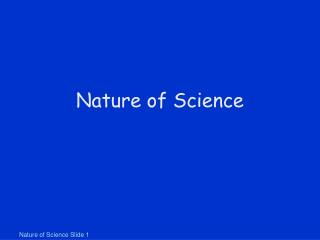# Nature of Science - PowerPoint PPT PresentationDownload PresentationNature of Science

Nature of Science
Download Presentation## Nature of Science

- - - - - - - - - - - - - - - - - - - - - - - - - - - E N D - - - - - - - - - - - - - - - - - - - - - - - - - - -
##### Presentation Transcript

1. Nature of Science

2. Scientific Measurements “No amount of experimentation can ever prove me right; a single experiment can prove me wrong.” ~Albert Einstein

3. Size of Earth • Eratosthenes measured the size of the earth in 235 BC • Calculated the circumference of earth by comparing shadows at local noon

4. Eratosthenes’ Method • Determine angle between sun’s rays and vertical • This angle is equal to the angle between the two radius vectors • 7.1o/360o = 1/50 • Distance between Syene and Alexandria known to be 800 km • 50*800 km = 40,000 km

5. Size of the Moon • Aristarchus determined the size of the moon in 240 BC • Eclipse of moon • Shadow of earth at moon’s distance has tapered by 1 moon diameter • Shadow was 2.5 X the moon’s diameter • Earth’s diameter is therefore 3.5 X moon’s diameter • Moon diameter = 2*6,370 km/3.5 = • 3640 km

6. Distance to Moon • Once Aristarchus had estimated the diameter of the moon (to within 5% of the present accepted value) • the above technique was used to calculate the distance to the moon • 110*3640 km = 400,400 km

7. Distance to Sun • Once earth-moon distance is known • Right triangle trigonometry allows the determination of the earth-sun distance • Aristarchus measured the angle X to be 87o • Actual value is 89.8o (difficult to measure) • Sun is 400 X farther than moon • Aristarchus estimated 20 X earth-moon distance

8. Size of the Sun • Pinhole camera • Sun’s diameter is • 150,000,000 km/110 • = 1,360,000 km

9. Pinhole Camera • Pinhole camera can be used to safely view a partial eclipse • Spaces between leaves act as pinholes during eclipse

10. Science Terms • Fact – a close agreement by competent observers – facts can change • Hypothesis – must be stated in a way that it can be disproved • Theory – a synthesis of a large body of information encompassing well-tested and verified hypotheses

11. Scientific Hypotheses • Scientific Hypothesis • Must be testable • Must be falsifiable • Our universe is surrounded by another, larger universe, with which we can have absolutely no contact. • Not testable • There are other inhabited planets in the universe. • Not falsifiable • The moon is made of green cheese. • Testable & Falsifiable

12. Check Yourself • Which of these is a scientific hypothesis? • Atoms are the smallest particles of matter that exist. • Space is permeated with an essence that is undetectable. • Albert Einstein was the greatest physicist of the twentieth century.

13. Check Yourself • Which of these is a scientific hypothesis? • Atoms are the smallest particles of matter that exist. • Space is permeated with an essence that is undetectable. • Albert Einstein was the greatest physicist of the twentieth century.

14. Scientific Method • There are many scientific methods – but generally • Recognize a question • Form a testable hypothesis • Make predictions • Test predictions • Formulate simplest general rule